MATLAB Function Referencebvp4c

Solve boundary value problems (BVPs) for ordinary differential equations

Syntax

• ```sol = bvp4c(odefun,bcfun,solinit)
sol = bvp4c(odefun,bcfun,solinit,options)
```

Arguments

 `odefun` A function handle that evaluates the differential equations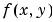. It can have the form```dydx = odefun(x,y) dydx = odefun(x,y,parameters) ``` where `x` is a scalar corresponding to, and `y` is a column vector corresponding to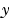. `parameters` is a vector of unknown parameters. The output `dydx` is a column vector. `bcfun` A function handle that computes the residual in the boundary conditions. For two-point boundary value conditions of the form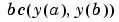, `bcfun` can have the form```res = bcfun(ya,yb) res = bcfun(ya,yb,parameters) ``` where `ya` and `yb` are column vectors corresponding to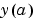and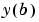. `parameters` is a vector of unknown parameters. The output `res` is a column vector.See Multipoint Boundary Value Problems for a description of bcfun for multipoint boundary value problems. `solinit` A structure containing the initial guess for a solution. You create `solinit` using the function `bvpinit`. `solinit` has the following fields. `x` Ordered nodes of the initial mesh. Boundary conditions are imposed at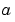= `solinit.x(1)` and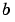= `solinit.x(end)`. `y` Initial guess for the solution such that `solinit.y(:,i)` is a guess for the solution at the node `solinit.x(i)`. `parameters` Optional. `A` vector that provides an initial guess for unknown parameters. The structure can have any name, but the fields must be named `x`, `y`, and `parameters`. You can form `solinit` with the helper function `bvpinit`. See `bvpinit` for details. `options` Optional integration argument. A structure you create using the `bvpset` function. See `bvpset` for details.

Description

```sol = bvp4c(odefun,bcfun,solinit) ``` integrates a system of ordinary differential equations of the form

•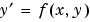on the interval [a,b] subject to two-point boundary value conditions

•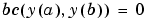`odefun` and `bcfun` are function handles. See Function Handles in the MATLAB Programming documentation for more information.

Parameterizing Functions Called by Function Functions, in the MATLAB mathematics documentation, explains how to provide additional parameters to the function `odefun`, as well as the boundary condition function `bcfun`, if necessary.

`bvp4c` can also solve multipoint boundary value problems. See Multipoint Boundary Value Problems. You can use the function `bvpinit` to specify the boundary points, which are stored in the input argument `solinit`. See the reference page for `bvpint` for more information.

The `bvp4c` solver can also find unknown parameters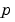for problems of the form

•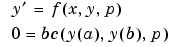wherecorresponds to `parameters`. You provide `bvp4c` an initial guess for any unknown parameters in `solinit.parameters`. The `bvp4c` solver returns the final values of these unknown parameters in `sol.parameters`.

`bvp4c` produces a solution that is continuous on `[a,b]` and has a continuous first derivative there. Use the function `deval` and the output `sol` of `bvp4c` to evaluate the solution at specific points `xint` in the interval `[a,b]`.

• ```sxint = deval(sol,xint)
```

The structure `sol` returned by `bvp4c` has the following fields:

 `sol.x` Mesh selected by `bvp4c` `sol.y` Approximation to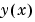at the mesh points of `sol.x` `sol.yp` Approximation to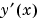at the mesh points of `sol.x` `sol.parameters` Values returned by `bvp4c` for the unknown parameters, if any `sol.solver` 'bvp4c'

The structure `sol` can have any name, and `bvp4c` creates the fields `x`, `y`, `yp`, `parameters`, and `solver`.

```sol = bvp4c(odefun,bcfun,solinit,options) ``` solves as above with default integration properties replaced by the values in `options`, a structure created with the `bvpset` function. See `bvpset` for details.

`solinit = bvpinit(x, yinit, params)` forms the initial guess `solinit` with the vector `params` of guesses for the unknown parameters.

Singular Boundary Value Problems

`bvp4c` solves a class of singular boundary value problems, including problems with unknown parameters `p`, of the form

•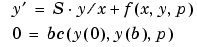The interval is required to be [0, b] with b > 0. Often such problems arise when computing a smooth solution of ODEs that result from partial differential equations (PDEs) due to cylindrical or spherical symmetry. For singular problems, you specify the (constant) matrix `S` as the value of the `'SingularTerm'` option of `bvpset`, and `odefun` evaluates only f(x, y, p). The boundary conditions must be consistent with the necessary condition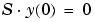and the initial guess should satisfy this condition.

Multipoint Boundary Value Problems

`bvp4c` can solve multipoint boundary value problems where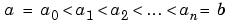are boundary points in the interval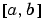. The points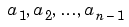represent interfaces that divideinto regions. `bvp4c` enumerates the regions from left to right (from a to b), with indices starting from 1. In region k,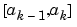, `bvp4c` evaluates the derivative as

• ```yp = odefun(x, y, k)
```

In the boundary conditions function

• ```bcfun(yleft, yright)
```

yleft(:, k) is the solution at the left boundary of. Similarly, `yright(:, k)` is the solution at the right boundary of region k. In particular,

• ```yleft(:, 1) = y(a)
```

and

• ```yright(:, end) = y(b)
```

For example, if there just one equation and the boundary points are 0 < 1 < 2, to specify the boundary conditions

•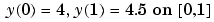•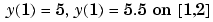`yleft` and `yright` have the following values.

• ```yleft = [4; 5];
yright = [4.5; 5.5];
```

The boundary condition function `bcfun` has the form

• ```function res = bc(yleft, yright)
res = [ yleft(1) - 4
yright(1) - 4.5
yleft(2) - 5
yright(2) - 5.5];
```

When you create an initial guess with

• ```solinit = bvpinit(xinit, yinit),
```

use double entries in `xinit` for each interface point. See the reference page for `bvpinit` for more information.

If `yinit` is a function, `bvpinit` calls `y = yinit(x, k)` to get an initial guess for the solution at `x` in region `k`. In the solution structure `sol` returned by `bpv4c`, `sol.x` has double entries for each interface point. The corresponding columns of `sol.y` contain the left and right solution at the interface, respectively.

For an example of solving a three-point boundary value problem, enter

• ```threebvp
```

Examples

Example 1. Boundary value problems can have multiple solutions and one purpose of the initial guess is to indicate which solution you want. The second order differential equation

•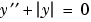has exactly two solutions that satisfy the boundary conditions

•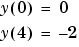Prior to solving this problem with `bvp4c`, you must write the differential equation as a system of two first order ODEs

•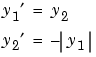Hereand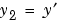. This system has the required form

•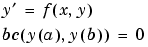The function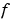and the boundary conditions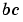are coded in MATLAB as functions `twoode` and `twobc`.

• ```function dydx = twoode(x,y)
dydx = [ y(2)
-abs(y(1))];

function res = twobc(ya,yb)
res = [ ya(1)
yb(1) + 2];
```

Form a guess structure consisting of an initial mesh of five equally spaced points in [0,4] and a guess of constant values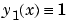and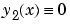with the command

• ```solinit = bvpinit(linspace(0,4,5),[1 0]);
```

Now solve the problem with

• ```sol = bvp4c(@twoode,@twobc,solinit);
```

Evaluate the numerical solution at 100 equally spaced points and plotwith

• ```x = linspace(0,4);
y = deval(sol,x);
plot(x,y(1,:));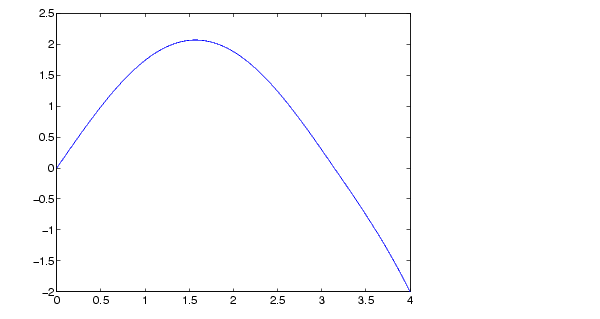```

You can obtain the other solution of this problem with the initial guess

• ```solinit = bvpinit(linspace(0,4,5),[-1 0]);
```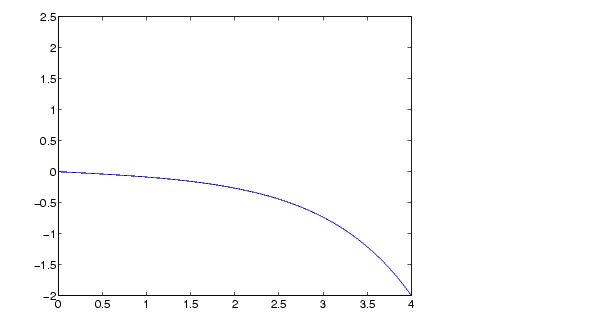Example 2. This boundary value problem involves an unknown parameter. The task is to compute the fourth (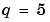) eigenvalue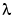of Mathieu's equation

•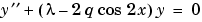Because the unknown parameteris present, this second order differential equation is subject to three boundary conditions

•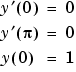It is convenient to use subfunctions to place all the functions required by `bvp4c` in a single M-file.

• ```function mat4bvp

lambda = 15;
solinit = bvpinit(linspace(0,pi,10),@mat4init,lambda);
sol = bvp4c(@mat4ode,@mat4bc,solinit);

fprintf('The fourth eigenvalue is approximately %7.3f.\n',...
sol.parameters)

xint = linspace(0,pi);
Sxint = deval(sol,xint);
plot(xint,Sxint(1,:))
axis([0 pi -1 1.1])
title('Eigenfunction of Mathieu''s equation.')
xlabel('x')
ylabel('solution y')
% ------------------------------------------------------------
function dydx = mat4ode(x,y,lambda)
q = 5;
dydx = [  y(2)
-(lambda - 2*q*cos(2*x))*y(1) ];
% ------------------------------------------------------------
function res = mat4bc(ya,yb,lambda)
res = [  ya(2)
yb(2)
ya(1)-1 ];
% ------------------------------------------------------------
function yinit = mat4init(x)
yinit = [  cos(4*x)
-4*sin(4*x) ];
```

The differential equation (converted to a first order system) and the boundary conditions are coded as subfunctions `mat4ode` and `mat4bc`, respectively. Because unknown parameters are present, these functions must accept three input arguments, even though some of the arguments are not used.

The guess structure `solinit` is formed with `bvpinit`. An initial guess for the solution is supplied in the form of a function `mat4init`. We chose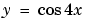because it satisfies the boundary conditions and has the correct qualitative behavior (the correct number of sign changes). In the call to `bvpinit`, the third argument (`lambda = 15`) provides an initial guess for the unknown parameter.

After the problem is solved with `bvp4c`, the field `sol.parameters` returns the value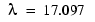, and the plot shows the eigenfunction associated with this eigenvalue.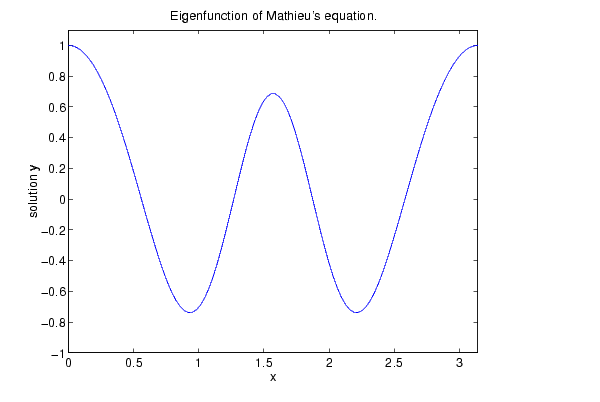Algorithms

`bvp4c` is a finite difference code that implements the three-stage Lobatto IIIa formula. This is a collocation formula and the collocation polynomial provides a C1-continuous solution that is fourth order accurate uniformly in `[a,b]`. Mesh selection and error control are based on the residual of the continuous solution.

See Also

`function_handle` (`@`), `bvpget`, `bvpinit`, `bvpset`, `deval`

References

  Shampine, L.F., M.W. Reichelt, and J. Kierzenka, "Solving Boundary Value Problems for Ordinary Differential Equations in MATLAB with bvp4c," available at `www.mathworks.com/bvp_tutorial`.

© 1994-2005 The MathWorks, Inc.